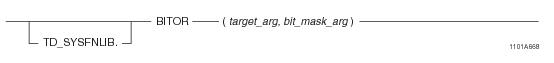## SQL Functions, Operators, Expressions, and Predicates

Product
Release Number
15.00
Language
English (United States)
Last Update
2018-09-24
dita:id
B035-1145
lifecycle
previous
Product Category

## BITOR

### Purpose

Performs the logical OR operation on the corresponding bits from the two input arguments.

### Syntaxwhere:

 Syntax element… Specifies… TD_SYSFNLIB the name of the database where the function is located. target_arg a numeric or variable byte expression. bit_mask_arg a fixed byte value, a variable byte value, or a numeric expression.

### ANSI Compliance

This is a Teradata extension to the ANSI SQL:2011 standard.

### Description

This function takes two bit patterns of equal length and performs the logical OR operation on each pair of corresponding bits as follows:

 IF... THEN the result is... either of the bits from the input arguments is 1 1 both of the bits from the input arguments are 0 0 any of the input arguments is NULL NULL

If the target_arg and bit_mask_arg arguments differ in length, the arguments are processed as follows:

• The target_arg and bit_mask_arg arguments are aligned on their least significant byte/bit.
• The smaller argument is padded with zeros to the left until it becomes the same size as the larger argument.
• For more information, see “Performing Bit-Byte Operations against Arguments with Non-Equal Lengths” on page 309.

### Argument Types and Rules

BITOR is an overloaded scalar function. The data type of the target_arg parameter can be one of the following:

• BYTEINT
• SMALLINT
• INTEGER
• BIGINT
• VARBYTE(n)
• The data type of the bit_mask_arg parameter varies depending upon the data type of the target_arg parameter. The following (target_arg, bit_mask_arg) input combinations are permitted:

 target_arg type bit_mask_arg type BYTEINT BYTE(1) BYTEINT BYTEINT SMALLINT BYTE(2) SMALLINT SMALLINT INTEGER BYTE(4) INTEGER INTEGER BIGINT BYTE(8) BIGINT BIGINT VARBYTE(n) VARBYTE(n)

The maximum supported size (n) for VARBYTE is 8192 bytes.

All expressions passed to this function must either match these declared data types or can be converted to these types using the implicit data type conversion rules that apply to UDFs. For example, BITOR(BYTEINT, INTEGER) is allowed because it can be implicitly converted to BITOR(INTEGER,INTEGER).

Note: The UDF implicit type conversion rules are more restrictive than the implicit type conversion rules normally used by Teradata Database. If any argument cannot be converted to one of the declared data types by following UDF implicit conversion rules, it must be explicitly cast. For details, see “Compatible Types” and “Parameter Types in Overloaded Functions” in SQL External Routine Programming.

If any argument cannot be converted to one of the declared data types, an error is returned indicating that no function exists that matches the DML UDF expression submitted.

### Result Type and Attributes

The result data type depends on the data type of the target_arg input argument that is passed to the function as shown in the following table:

 IF the data type of target_arg is... THEN the result type is... AND the result format is the default format for... BYTEINT BYTEINT BYTEINT SMALLINT SMALLINT SMALLINT INTEGER INTEGER INTEGER BIGINT BIGINT BIGINT VARBYTE(n) VARBYTE(n) VARBYTE(n)

The maximum supported size (n) for VARBYTE is 8192 bytes.

The default title for BITOR is: BITOR(target_arg, bit_mask_arg).

For information on default data type formats, see SQL Data Types and Literals.

### Example

In the following query, the input argument 23 has a data type of BYTEINT and a binary representation of 00010111. The input argument 45 has a data type of BYTEINT and a binary representation of 00101101. The bitwise OR product of the two arguments results in a BYTEINT value of 63, or binary 00111111, which is returned by the query.

`   SELECT BITOR(23,45);`